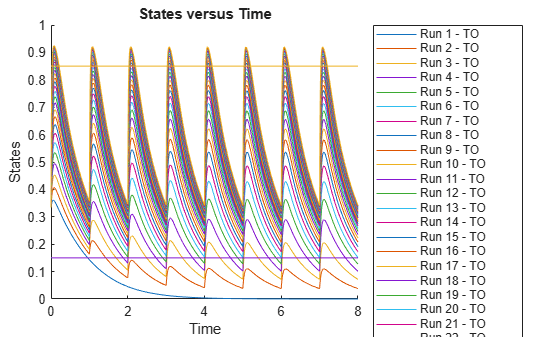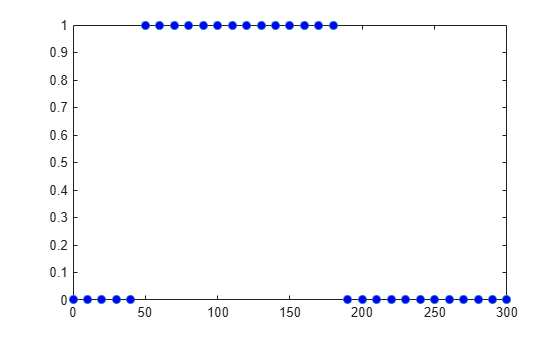# updateobservable

Update observable expressions or units in SimData

## Syntax

``sdout = updateobservable(sdin,obsNames,obsExpressions)``
``sdout = updateobservable(sdin,obsNames,obsExpressions,'Units',units)``
``sdout = updateobservable(sdin,obsNames,'Units',units)``

## Description

example

````sdout = updateobservable(sdin,obsNames,obsExpressions)` returns a new `SimData` object (or array of objects) `sdout` after copying the input `SimData` `sdin` and recalculating the observables using updated expressions. `obsNames` and `obsExpressions` are the existing observable names and their corresponding expressions to update, respectively. The number of expressions must match the number of observable names.```

example

````sdout = updateobservable(sdin,obsNames,obsExpressions,'Units',units)` recalculates the observables `obsNames` using the updated expressions `obsExpressions` and the specified `units`. The number of units must match the number of observable names.```

example

````sdout = updateobservable(sdin,obsNames,'Units',units)` recalculates the observables `obsNames` using the specified `units`. The number of units must match the number of observable names.```

## Examples

collapse all

`sbioloadproject tmdd_with_TO.sbproj`

Set the target occupancy (`TO`) as a response.

```cs = getconfigset(m1); cs.RuntimeOptions.StatesToLog = 'TO';```

Get the dosing information.

`d = getdose(m1,'Daily Dose');`

Scan over different dose amounts using a `SimBiology.Scenarios` object. To do so, first parameterize the `Amount` property of the dose. Then vary the corresponding parameter value using the `Scenarios` object.

```amountParam = addparameter(m1,'AmountParam','Units',d.AmountUnits); d.Amount = 'AmountParam'; d.Active = 1; doseSamples = SimBiology.Scenarios('AmountParam',linspace(0,300,31));```

Create a `SimFunction` to simulate the model. Set `TO `as the simulation output.

```% Suppress informational warnings that are issued during simulation. warning('off','SimBiology:SimFunction:DOSES_NOT_EMPTY'); f = createSimFunction(m1,doseSamples,'TO',d)```
```f = SimFunction Parameters: Name Value Type Units _______________ _____ _____________ ____________ {'AmountParam'} 1 {'parameter'} {'nanomole'} Observables: Name Type Units ______ _____________ _________________ {'TO'} {'parameter'} {'dimensionless'} Dosed: TargetName TargetDimension Amount AmountValue AmountUnits _______________ ___________________________________ _______________ ___________ ____________ {'Plasma.Drug'} {'Amount (e.g., mole or molecule)'} {'AmountParam'} 1 {'nanomole'} TimeUnits: day ```
`warning('on','SimBiology:SimFunction:DOSES_NOT_EMPTY');`

Simulate the model using the dose amounts generated by the `Scenarios` object. In this case, the object generates 31 different doses; hence the model is simulated 31 times and generates a `SimData` array.

```doseTable = getTable(d); sd = f(doseSamples,cs.StopTime,doseTable)```
``` SimBiology Simulation Data Array: 31-by-1 ModelName: TMDD Logged Data: Species: 0 Compartment: 0 Parameter: 1 Sensitivity: 0 Observable: 0 ```

Plot the simulation results. Also add two reference lines that represent the safety and efficacy thresholds for `TO`. In this example, suppose that any `TO` value above 0.85 is unsafe, and any `TO` value below 0.15 has no efficacy.

```h = sbioplot(sd); time = sd(1).Time; h.NextPlot = 'add'; safetyThreshold = plot(h,[min(time), max(time)],[0.85, 0.85],'DisplayName','Safety Threshold'); efficacyThreshold = plot(h,[min(time), max(time)],[0.15, 0.15],'DisplayName','Efficacy Threshold');```Postprocess the simulation results. Find out which dose amounts are effective, corresponding to the `TO` responses within the safety and efficacy thresholds. To do so, add an observable expression to the simulation data.

```% Suppress informational warnings that are issued during simulation. warning('off','SimBiology:sbservices:SB_DIMANALYSISNOTDONE_MATLABFCN_UCON'); newSD = addobservable(sd,'stat1','max(TO) < 0.85 & min(TO) > 0.15','Units','dimensionless')```
``` SimBiology Simulation Data Array: 31-by-1 ModelName: TMDD Logged Data: Species: 0 Compartment: 0 Parameter: 1 Sensitivity: 0 Observable: 1 ```

The addobservable function evaluates the new observable expression for each `SimData` in `sd` and returns the evaluated results as a new `SimData` array, `newSD`, which now has the added observable (`stat1`).

SimBiology stores the observable results in two different properties of a `SimData` object. If the results are scalar-valued, they are stored in `SimData.ScalarObservables`. Otherwise, they are stored in `SimData.VectorObservables`. In this example, the `stat1` observable expression is scalar-valued.

Extract the scalar observable values and plot them against the dose amounts.

```scalarObs = vertcat(newSD.ScalarObservables); doseAmounts = generate(doseSamples); figure plot(doseAmounts.AmountParam,scalarObs.stat1,'o','MarkerFaceColor','b')```The plot shows that dose amounts ranging from 50 to 180 nanomoles provide `TO` responses that lie within the target efficacy and safety thresholds.

You can update the observable expression with different threshold amounts. The function recalculates the expression and returns the results in a new `SimData` object array.

`newSD2 = updateobservable(newSD,'stat1','max(TO) < 0.75 & min(TO) > 0.30');`

Rename the observable expression. The function renames the observable, updates any expressions that reference the renamed observable (if applicable), and returns the results in a new `SimData` object array.

`newSD3 = renameobservable(newSD2,'stat1','EffectiveDose');`

Restore the warning settings.

`warning('on','SimBiology:sbservices:SB_DIMANALYSISNOTDONE_MATLABFCN_UCON');`

## Input Arguments

collapse all

Input simulation data, specified as a `SimData` object or array of objects.

Names of existing observable expressions, specified as a character vector, string, string vector, or cell array of character vector.

Example: `{'max_drug','mean_drug'}`

Data Types: `char` | `string` | `cell`

Observable expressions, specified as a character vector, string, string vector, or cell array of character vectors. The number of expressions must match the number of observable names.

Example: `{'max(drug)','mean(drug)'}`

Data Types: `char` | `string` | `cell`

Units for the observable expressions, specified as a character vector, string, string vector, or cell array of character vectors. The number of units must match the number of observable names.

Example: `{'nanomole/liter','nanomole/liter'}`

Data Types: `char` | `string` | `cell`

## Output Arguments

collapse all

Simulation data with observable results, returned as a `SimData` object or array of objects.

## Version History

Introduced in R2020a